# SAFETY

Let Q be a set of queries that are all either count queries or sum queries with the same summary attribute. Loosely speaking, given a sensitivity criterion, Q is considered "safe" by the query-answering system if every sensitive information contained in Q is protected. We now make this notion precise. First of all, it should be noted that most sensitivity criteria—including the n threshold rule and the (n, k%) dominance rule—can be expressed by means of a linear subadditive function defined on the powerset of the underlying population I and called a sensitivity function (Cox, 1981), and a query Q is sensitive according to the to the criterion specified by the sensitivity function σ if σ(I[Q]) > 0. For example, the (n, k%) dominance rule for sum queries with summary attribute a is specified by the sensitivity function σ which in correspondence of a subset J of I takes the value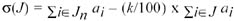where ai is the value of a for individual i, and Jn = J if |J| n and Jn is the set of the n individuals with largest values of a otherwise.

By the subadditivity of the function σ, if J and J are two subsets of I, then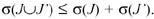Remark 1: Let Q, Q , and Q′′ be queries such that I[Q] = I[Q] I[Q′′]. Then, if neither Q nor Q′′ is sensitive, then Q is not sensitive; on the other hand, if Q is sensitive, then Q or Q′′ is sensitive.

Consider now a set of queries Q and let (H, q) be the answer map of Q. Let Q* be the closure of Q, and let Qσ be the set of sensitive queries Q(C[F]) for some subset F of E; that is,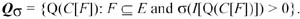We say that Q is strongly safe if

Qσ Q* = Ø

We now introduce a subset of Q* which is sufficient to assure strong safety. Recall that the queries in Q* correspond to the sets F of edges of H for which the sum expression Σe F x(e) is a D-invariant. Let F* be the family of such subsets of E and let F+ be the subfamily of F* formed by minimal (with respect to set-inclusion) nonempty members of F*. From the very definition of a D-invariant sum expression, it follows that F* is closed under disjoint union and proper difference, which implies that every nonempty member of F* is the disjoint union of one or more members of F+. Let Q+ be the set of queries in Q* that correspond to edge sets in F+. Of course, if Q is strongly safe, then Qσ Q+ = Ø. On the other hand, if Q is not strongly safe, then there exists a query Q(C[F]) in Qσ such that F belongs to F*. Since F cannot be empty, there is a subset F of F that belongs to F+ and, by Remark 1, is such that Q(C[F]) is in Qσ which proves that Qσ Q+ Ø. To sum up, Q is strongly safe if and only if Qσ Q+ = Ø.

The following is a weaker condition than strong safety which is often used in the security analysis of statistical tables. A query set Q is weakly safe if no elementary query in Q* is sensitive. Of course, if Q is strongly safe, then Q is also weakly safe; but, the converse need not hold as shown by the following example.

Example 9: Let the graph shown in Figure 31 be the hypergraph associated with a set Q of four additive real-valued queries.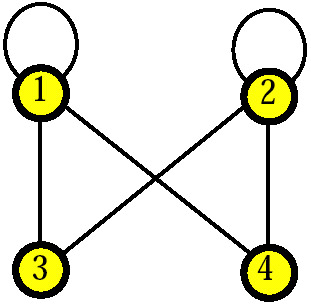Figure 31

Q is weakly safe since no elementary query belongs to the closure of Q. However, if the query Q corresponding to the pair F of self-loops {1} and {2} were sensitive, then Q would be not strongly safe because Q belongs to the closure of Q (the incidence vector of F can be written as a linear combination of the rows of the incidence matrix of the graph).

The previous two notions of safety are related to exact inference. We now introduce their counterparts when approximate inference is taken into account. To this end, consider any query Q corresponding to some subset of E, and let q be the value of Q. By the protection interval for Q, we mean the intersection of D with the real interval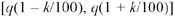for a fixed percentage k.

A query set Q is strongly safe with respect to the k% rule (strongly k-safe, for short) if, for each sensitive query Q corresponding to a set of edges of H, the feasibility range for the value of Q strictly contains the protection interval for Q. Note that Q is strongly safe if and only if Q is strongly 0-safe; furthermore, when k > 0, if Q is strongly k-safe, then Q is strongly safe, but the converse need not hold.

A query set Q is weakly safe with respect to the k% rule (weakly k-safe, for short) if, for each sensitive elementary query Q, the feasibility range for the value of Q strictly contains the protection interval for Q. Note that Q is weakly safe if and only if Q is weakly 0-safe; furthermore, when k > 0, if Q is weakly k-safe, then Q is weakly safe, but the converse need not hold.

At this point, the following question naturally arises: how can the query-answering system test Q for safety? In the next two subsections, we answer this question by considering the four above safety criteria in the cases that the queries in Q are additive queries with values in and in. The safety tests we present make use of an edge-weighted hypergraph (H, w), we call the data map of Q, where H is the hypergraph associated with Q and, for each edge e of H, the weight w(e) of e is given by the value of the elementary query corresponding to e. Moreover, each edge e of H is labeled by the corresponding class of the characteristic partition of the active domain of the category variable. Finally, an edge e of H is marked (sensitive) if and only if the corresponding elementary query is sensitive. Note that, by Remark 1, every edge set corresponding to a sensitive query must contain at least one marked edge.

Example 2 (continued): The data map (H, w) of Q = {Q1, Q2, Q3} is shown in Figure 32. The weights of the edges of H are w(e1) = 15.0, w(e2) = 9.0, and w(e3) = 6.0; moreover, the labels of e1, e2, and e3 are {Direction}, {Administration}, and {Services}, respectively. Finally, only the edge e1 is marked (*).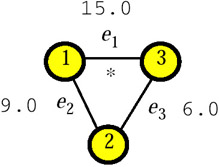Figure 32: A Data Map.

The Case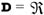Testing Q for weak safety. Let InvH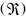be the set of edges of H corresponding to elementary queries in the closure of Q. The test for weak safety consists in determining the set InvHand, if no edge in InvHis marked, then and only then is Q recognized to be weakly safe.

The set InvHcan be determined by checking, for each edge e of H, the consistency of equation system (3) where f is taken to be the incidence vector of the singleton {e}. However, if Q is graphical, there is a linear algorithm for determining InvH(Malvestuto & Mezzini, 2002), which is based on the following property: an edge of H belongs to Invif and only if its removal increases the number of bipartite components. It is easily seen that the removal of an edge e from a connected graph H increases the number of bipartite components if and only if either e participates in all odd cycles, or e is a cut edge and at least one of the two subgraphs of H separated by e is bipartite. (Note that, if H is bipartite, then Invcoincides with the set of cut edges.) Since both the set of edges of H that participate in all odd cycles and the set of cut edges separating bipartite subgraphs of H can be determined in linear time (Malvestuto & Mezzini, 2002), the problem of testing Q for weak safety can be solved in linear time.

Testing Q for strong safety. The family of edge sets in F+ is first determined. If for each set F in F+, the query corresponding to F is not sensitive, then and only then is Q recognized to be strongly safe.

An open problem is the existence of an effective procedure for determining F+ without examining all sets of edges of H. What is known is only a graphical characterization of the sets in F+ when Q is graphical (Malvestuto & Mezzini, 2001), which entails that if H is bipartite, then F+ consists of all and the only simple bonds of H (see Figure 3). Unfortunately, as shown below, the number of simple bonds of a bipartite graph can be exponential in the numbers of its edges, which entails that the size of F+ may be exponential in the size of H.

Example 10: Let H be the bipartite (connected) graph shown in Figure 33. Note that H contains O(k2) vertices and O(k2) edges.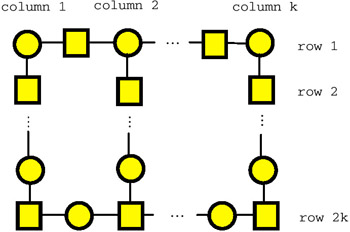Figure 33

Let F be any set of k edges of the form shown in Figure 34Figure 34

one for each of the k columns. It is easily seen that F is a simple bond of H, and that the number of such simple bonds is equal to kk.

The Case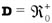Testing Q for weak safety. Let InvH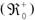be the set of edges of H corresponding to elementary queries in the closure of Q. The test for weak safety consists in determining the set InvH, and if no edge in InvHis marked, then and only then is Q recognized to be weakly safe. Since InvH= kerne(H) InvH, where H = Hkernel(H), if Q is graphical then both kernel(H) and InvHcan be determined in linear time from the data map of Q and, hence, the problem of testing Q for weak safety can be solved in linear time.

Testing Q for strong safety. The test of strong safety is the same as in the caseafter determining kernel(H), which is computed as above.

Testing Q for weak k-safety. For each marked edge e of H, the feasibility range for the value of the elementary query corresponding to e is determined. If for each marked edge e of H, the feasibility range for the value of the elementary query corresponding to e strictly contains the protection interval for the elementary query corresponding to e, then and only then is Q recognized to be weakly k-safe. We saw that if Q is graphical, then the feasibility range for the value of an elementary query can be determined in cubic time so that testing Q for weak k-safety requires a polynomial time.

Testing Q for strong k-safety. The family Fσ of the edge sets of H to which correspond sensitive queries is first determined. Next, for each F in Fσ, the feasibility range for the value of the query corresponding to F is determined and compared with its protection interval. If, for each F in Fσ, the feasibility range strictly contains the protection interval, then and only then is Q recognized to be strongly k-safe.

The existence of a procedure for determining Fσ without examining all the edge sets in H is an open problem.Multidimensional Databases: Problems and Solutions
ISBN: 1591400538
EAN: 2147483647
Year: 2003
Pages: 150